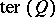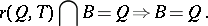# Tertiary ideal

(diff) ← Older revision | Latest revision (diff) | Newer revision → (diff)

An idealof a ringwhich cannot be expressed as the intersection of a right fractional idealand an ideal, each strictly larger than. All irreducible ideals are tertiary. In Noetherian rings, tertiary ideals are the same as primary ideals (cf. Additive theory of ideals; Primary ideal; Primary decomposition).

Suppose that the ringsatisfies the maximum condition for left and right fractional ideals, and that every ideal decomposes as an intersection of finitely many indecomposable ideals. Then for every idealthere exists a tertiary radical,, the largest idealofsuch that, for any ideal,As for primary ideals, the intersection theorem, the existence theorem and the uniqueness theorem are true for tertiary ideals.

An analysis of the properties of left and right fractions (of ideals of a ring, of submodules of a module, and others) leads to systems with fractions in which the general notions of-primarity and-primary radicals occur naturally. This allows one to formulate the "intersection" , "existence" and "uniqueness theorems" as axioms. In this approach, tertiarity is the unique notion of primarity for which all these three theorems hold, i.e. it is the unique "good" generalization of classical primarity (cf. , ).

How to Cite This Entry:
Tertiary ideal. Encyclopedia of Mathematics. URL: http://encyclopediaofmath.org/index.php?title=Tertiary_ideal&oldid=13632
This article was adapted from an original article by V.A. Andrunakievich (originator), which appeared in Encyclopedia of Mathematics - ISBN 1402006098. See original article